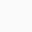### How to use if else, while and for loops in python?

•• 1

The if and else clause are used to structure our code with checks for conditions. The if statement is followed by an expression that we use to express a condition, and delimiters the so-called if block. The block is executed if and only if the expression evaluates to True.

Example 1:

By the way, even though in the example here, we are adding an else block, remember that an else block is optional.

We may have a case with more than one alternative conditional block to write. For such cases, we use elif for each of them except for the last one, for which we use the else clause.

Here is an example using if, elif (twice), and else:

Example 2:

For each execution, depending on the condition which is true, the corresponding (if or elif) code block is executed, which ends the entire if block, otherwise, if an else block exists, it is executed.

The if statement is very powerful since what we are doing most of the time is making decisions based on conditions.

The while loop:

A so-called while loop specifies a condition and a block of code to be executed until the condition evaluates to False or a break statement, which we will cover later, is encountered.

Here is what happens with this piece of code: unless the condition of the while statement (x < 20) becomes false, the code within the loop is executed. The check for the condition being true is done again and again until loop terminates. And our variable is incremented by 2 at each step of the loop, so at some point, x takes the value of 20 and the condition becomes false, which makes the loop to terminates.

Since a loop is based on a condition being true, guess what happens if the condition never becomes false: we get an infinite loop. Let’s take the following code:

As an interesting test that if you execute this piece of code you will se that if produces infinite loop, continuously printing the number 1.

To avoid the infinite loop, a better code would be as follows:

The for loop:

The for loop is another way to iterate over a sequence. It goes through each item is a sequence.

The for statement uses the in keyword for walking the sequences. You may also want to think of it as the for… in statement. Here is a simple example:

As the output shows, for every element in the string (a text character), we print it. The variable I is called the loop variable. The block of code delimited by the for statement executes once for each item in the sequence (which is also called the iterable).

In some cases, we want to build a new sequence, while iterating over the current one, using each item we have at hand. For example, building on the previous example we may want to build a new string which contains the same letters but where the D is capitalized.

## Popular Posts

1.dualism says: